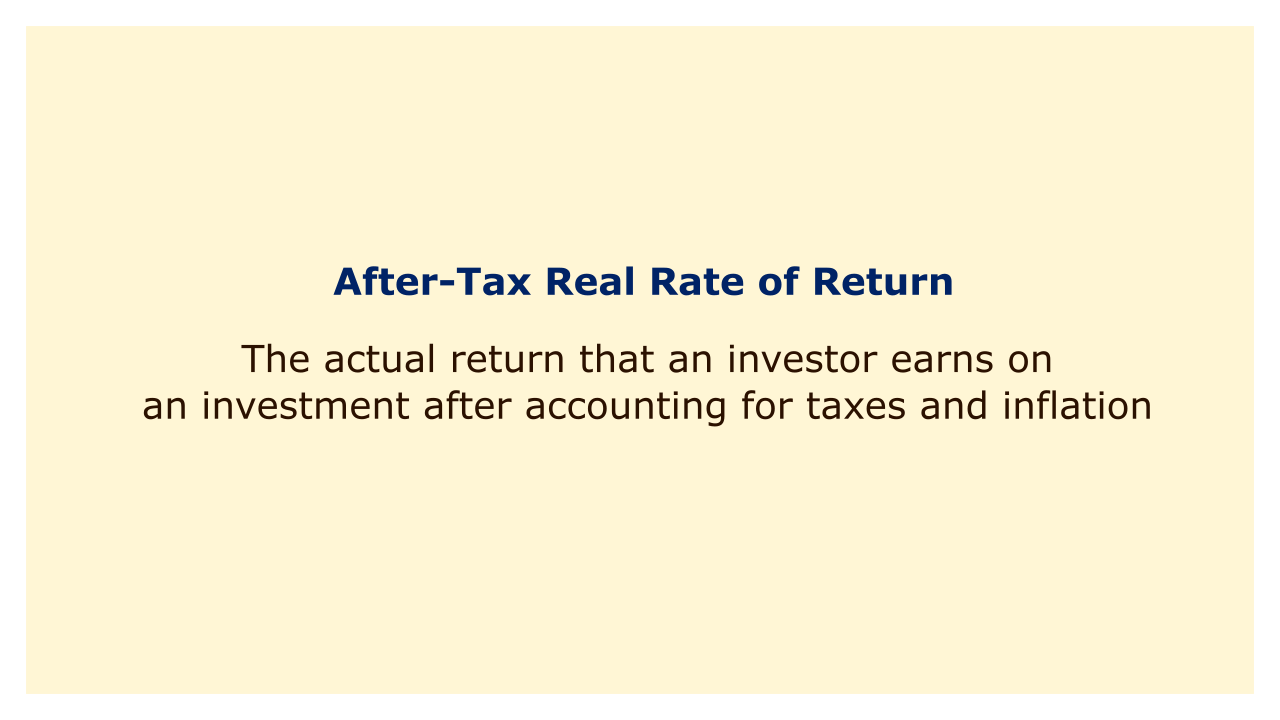# After-Tax Real Rate of ReturnImage: Moneybestpal.com

### The after-tax real rate of return is among the key ideas in investing. This is the real return on investment that an investor receives after deducting taxes and inflation.

The after-tax real rate of return can be calculated as follows:

After-tax real rate of return = [(1 + nominal rate of return) x (1 - tax rate)] - 1 - inflation rate

The nominal rate of return is the percentage increase or decrease in the value of an investment before taxes and inflation. The tax rate is the percentage of the investment income that is paid to the government as taxes. The inflation rate is the percentage increase in the general level of prices over a period of time.

For example, suppose an investor buys a bond that pays 5% interest per year and sells it after one year for the same price. The investor's nominal rate of return is 5%. If the investor's tax rate is 25% and the inflation rate is 2%, then the investor's after-tax real rate of return is:

After-tax real rate of return = [(1 + 0.05) x (1 - 0.25)] - 1 - 0.02

After-tax real rate of return = 0.0175 or 1.75%

This means that the investor's purchasing power has increased by 1.75% after one year, after accounting for taxes and inflation.

The nominal rate of return does not accurately reflect the actual change in the investor's wealth, hence the after-tax real rate of return is a better indicator of an investment's profitability. Taxes and inflation have the potential to reduce an investment's value over time, but they are not taken into account by the nominal rate of return.

Comparing investments with various tax structures and inflation concerns can also be done using the after-tax real rate of return. As an illustration, let's say that an investor has two choices: a municipal bond that is tax-exempt and pays 4% interest annually, or a taxable bond that pays 6% interest annually. If the investor's tax rate is 25% and the inflation rate is 2%, then the after-tax real rates of return for both bonds are:

After-tax real rate of return for taxable bond = [(1 + 0.06) x (1 - 0.25)] - 1 - 0.02

After-tax real rate of return for taxable bond = 0.0225 or 2.25%

After-tax real rate of return for tax-exempt bond = [(1 + 0.04) x (1 - 0)] - 1 - 0.02

After-tax real rate of return for tax-exempt bond = 0.02 or 2%

In this instance, while having a lower nominal rate of return, the taxable bond has a higher after-tax real rate of return than the tax-exempt bond. This is owing to the fact that the tax-exempt bond's lower interest income does not entirely make up for the inflation-related loss of purchasing power, whereas the taxable bond's greater interest income more than makes up for the taxes that were paid on it.

In order to make well-informed selections that maximize one's wealth and financial goals, it is crucial to take into account alternative assets' after-tax real rates of return rather than their nominal rates of return.
Tags